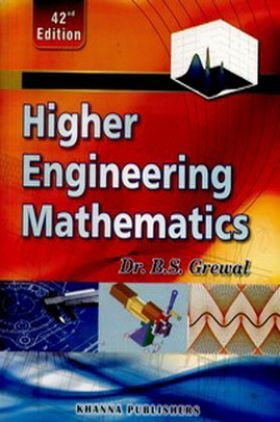# Higher Engineering Mathematics

14225 Views

This product is currently not available.

Save extra with 1 Offers

Get ₹ 50

Instant Cashback on the purchase of ₹ 400 or above

Product Specifications

 Publisher Faculty Notes All Engineering 2nd SEM books by Faculty Notes Available Available in all digital devicesHIGHER ENGINEERING MATHEMATICS

The book provides a clear exposition of essential tools of applied mathematics from a modern point of view and meets complete requirements of engineering and computer science students. Every effort has been made to keep the presentation at once simple and lucid. It is written with the firm conviction that a good book is one that can be read with minimum guidance from the instructor. To achieve this, more than the usual number of solved examples, followed by properly graded problems have been given. Many of the examples and problems have been selected from recent papers of various university and other engineering examinations. Basic Concepts and Useful Information has been given in an Appendix. However, the subject matter has been set in eight main units:

Unit I: Algebra, Vectors and Geometry
Solution of Equations
Linear Algebra: Determinants, Matrices
Vector Algebra and Solid Geometry

Unit II: Calculus
Differential Calculus & Its Applications
Partial Differentiation & Its Applications
Integral Calculus & Its Applications
Multiple Integrals & Beta, Gamma Functions
Vector Calculus & Its Applications

Univ III: Series
Infinite Series
Fourier Series & Harmonic Anslysis

Unit IV: Differential Equations
Differential Equations of First Order
Applications of Differential Equations of First Order
Linear Differential Equations
Applications of Linear Differential Equations
Differential Equations of Other Types
Series Solution of Differential Equations and Special Functions
Partial Differential Equations
Applications of Partial Differential Equations

Unit V: Complex Analysis
Complex Numbers and Functions
Calculus of Complex Functions

Unit VI: Transforms
Laplace Transforms
Fourier Transforms
Z-Transforms

Unit VII: Numerical Techniques
Empirical Laws and Curve-fitting
Statistical Methods
Probability and Distributions
Sampling and Inference
Numerical Solution of Equations
Finite Differences and Interpolation
Numerical Differentiation and Integration
Difference Equations
Numerical Solution of Ordinary Differential Equations
Numerical Solution of Partial Differential Equations
Linear Programming

Unit VIII: Special Topics
Calculus of Variations
Integral Equations
Discrete Mathematics
Tensor Analysis
Useful Information
Tables